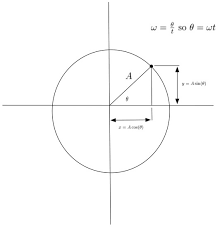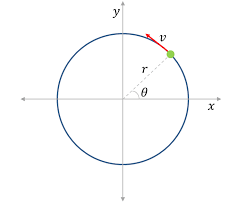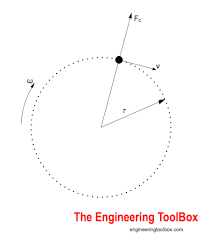## How to Calculate and Solve for Maximum Velocity to avoid Overturning of a Vehicle moving along a Level Circular Path | The Calculator EncyclopediaThe image above represents the maximum velocity to avoid overturning of a vehicle moving along a level circular path.

To compute for the maximum velocity, four essential parameters are needed and these parameters are Acceleration due to Gravity (g), Height of Centre of Gravity of the Vehicle from Ground Level (h), Radius of Circular Path (r) and Half of the Distance between the Centre Lines of the Wheel (a).

The formula for calculating the maximum velocity:

vmax = √(gra / h)

Where:
vmax = Maximum Velocity to avoid Overturning of a Vehicle moving along a Level Circular Path
g = Acceleration due to Gravity
h = Height of Centre of Gravity of the Vehicle from Ground Level
r = Radius of Circular Path
a = Half of the Distance between the Centre Lines of the Wheel

Let’s solve an example;
Find the maximum velocity when the Acceleration due to Gravity (g) is 10.2, Height of Centre of Gravity of the Vehicle from Ground Level (h) is 14, Radius of Circular Path (r) is 22 and Half of the Distance between the Centre Lines of the Wheel (a) is 32.

This implies that;
g = Acceleration due to Gravity = 10.2
h = Height of Centre of Gravity of the Vehicle from Ground Level = 14
r = Radius of Circular Path = 22
a = Half of the Distance between the Centre Lines of the Wheel = 32

vmax = √(gra / h)
vmax = √((10.2)(22)(32)/14)
vmax = √((7180.79)/14)
vmax = √(512.91)
vmax = 22.647

Therefore, the maximum velocity to avoid Overturning of a Vehicle moving along a Level Circular Path is 22.647 m/s.

## How to Calculate and Solve for the Reaction at the Inner Wheel of a Vehicle moving along a Level Circular Path | The Calculator EncyclopediaThe image represents reaction at the inner wheel of a vehicle moving along a level circular path.

To compute for the reaction, six essential parameters are needed and these parameters are Mass of the Vechicle (m), Acceleration due to Gravity (g), Velocity of the Vehicle (v), Height of Centre of Gravity of the Vehicle from Ground Level (h), Radius of Circular Path (r) and Half of the Distance between the Centre Lines of the Wheel (a).

The formula for calculating the reaction at the inner wheel of a vehicle moving along a level circular path:

RA = mg / 2[1 – v²h / gra]

Where:
RA = Reaction at the Inner Wheel of a Vehicle moving along a Level Circular Path
m = Mass of the Vechicle
g = Acceleration due to Gravity
v = Velocity of the Vehicle
h = Height of Centre of Gravity of the Vehicle from Ground Level
r = Radius of Circular Path
a = Half of the Distance between the Centre Lines of the Wheel

Let’s solve an example;
Find the reaction when Mass of the Vechicle (m) is 13, Acceleration due to Gravity (g) is 9.8, Velocity of the Vehicle (v) is 11, Height of Centre of Gravity of the Vehicle from Ground Level (h) is 5, Radius of Circular Path (r) is 7 and Half of the Distance between the Centre Lines of the Wheel (a) is 3.

This implies that;
m = Mass of the Vechicle = 13
g = Acceleration due to Gravity = 9.8
v = Velocity of the Vehicle = 11
h = Height of Centre of Gravity of the Vehicle from Ground Level = 5
r = Radius of Circular Path = 7
a = Half of the Distance between the Centre Lines of the Wheel = 3

RA = mg / 2[1 – v²h / gra]
RA = 13(9.8) / 2[1 – (11)²(5) / (9.8)(7)(3)]
RA = 127.4 / 2[1 – (121)(5) / 205.8]
RA = 63.7[1 – 605 / 205.8]
RA = 63.7[1 – 2.939]
RA = 63.7[-1.939]
RA = -123.56

Therefore, the reaction at the inner wheel of a vehicle moving along a level of circular path is -123.56 N.

## How to Calculate and Solve for the Reaction at the Outer Wheel of a Vehicle moving along a Level Circular Path | Nickzom CalculatorThe image represents reaction at the outer wheel of a vehicle moving along a level circular path.

To compute for the reaction, six essential parameters are needed and these parameters are Mass of the Vechicle (m), Acceleration due to Gravity (g), Velocity of the Vehicle (v), Height of Centre of Gravity of the Vehicle from Ground Level (h), Radius of Circular Path (r) and Half of the Distance between the Centre Lines of the Wheel (a).

The formula for calculating the reaction at the outer wheel of a vehicle moving along a level circular path:

RB = mg / 2[1 + v²h/gra]

Where;
RB = Reaction at the Outer Wheel of a Vehicle moving along a Level Circular Path
m = Mass of the Vechicle
g = Acceleration due to Gravity
v = Velocity of the Vehicle
h = Height of Centre of Gravity of the Vehicle from Ground Level
r = Radius of Circular Path
a = Half of the Distance between the Centre Lines of the Wheel

Let’s solve an example;
Find the reaction when Mass of the Vechicle (m) is 12, Acceleration due to Gravity (g) is 9.8, Velocity of the Vehicle (v) is 28, Height of Centre of Gravity of the Vehicle from Ground Level (h) is 16, Radius of Circular Path (r) is 8 and Half of the Distance between the Centre Lines of the Wheel (a) is 4.

This implies that;
m = Mass of the Vechicle = 12
g = Acceleration due to Gravity = 9.8
v = Velocity of the Vehicle = 28
h = Height of Centre of Gravity of the Vehicle from Ground Level = 16
r = Radius of Circular Path = 8
a = Half of the Distance between the Centre Lines of the Wheel = 4

RB = mg / 2[1 + v²h/gra]
RB = 12 x 9.8 / 2[1 + 28² x 16/9.8 x 8 x 4]
RB = 117.60 / 2[1 + 784 x 16/313.6]
RB = 58.80[1 + 40]
RB = 58.80
RB = 58.80
RB = 2410.8

Therefore, the reaction of the outer wheel of a vehicle moving along a level circular path is 2410.8 N.